Courses

# Test: Basic Geometrical Ideas

## 10 Questions MCQ Test Mathematics (Maths) Class 6 | Test: Basic Geometrical Ideas

Description
This mock test of Test: Basic Geometrical Ideas for Class 6 helps you for every Class 6 entrance exam. This contains 10 Multiple Choice Questions for Class 6 Test: Basic Geometrical Ideas (mcq) to study with solutions a complete question bank. The solved questions answers in this Test: Basic Geometrical Ideas quiz give you a good mix of easy questions and tough questions. Class 6 students definitely take this Test: Basic Geometrical Ideas exercise for a better result in the exam. You can find other Test: Basic Geometrical Ideas extra questions, long questions & short questions for Class 6 on EduRev as well by searching above.
QUESTION: 1

### How many points does the given figure has?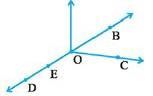Solution:

(B,C,D,E,O)5 POINTS

QUESTION: 2

### In the given figure, the ray will be named as _____.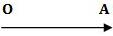Solution:

We start from the letter which has an exact point (O here) and then we write the letter which is extended infinitely (A here) hence OA

QUESTION: 3

### Which of the following is an open curve?

Solution:

Rest of the figures are totally closed but only figure D is open .

QUESTION: 4

The line segment forming a polygon are called _________________.

Solution:

The line segments that form a polygon are called the sides of the polygon. A point where two sides meet is a vertex (plural form is vertices).

QUESTION: 5

Two distinct lines meeting at a points are called _____________.

Solution:

Intersecting Lines: Two lines which meet at one point are said to be intersecting lines. The common point is called the ‘point of intersection’.

QUESTION: 6

How many lines pass through one given point?

Solution:

Countless or Infinite number of lines can pass through a single point.

QUESTION: 7

Name the point intersection in the given figure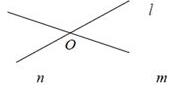Solution:

These two lines meet at point O and hence it is called the intersection point of the two lines.

QUESTION: 8

How many lines pass through two given points?

Solution:

Only one line can pass through two given points.

QUESTION: 9

Which of the following is pair of opposite sides in the given figure?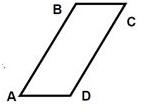Solution:
QUESTION: 10

Which of the following is the pair of adjacent angles in the given figure ?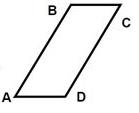Solution:

The angles which have a ray in common are called adjacent angles. Here A and B have AB as common and hence they are adjacent.